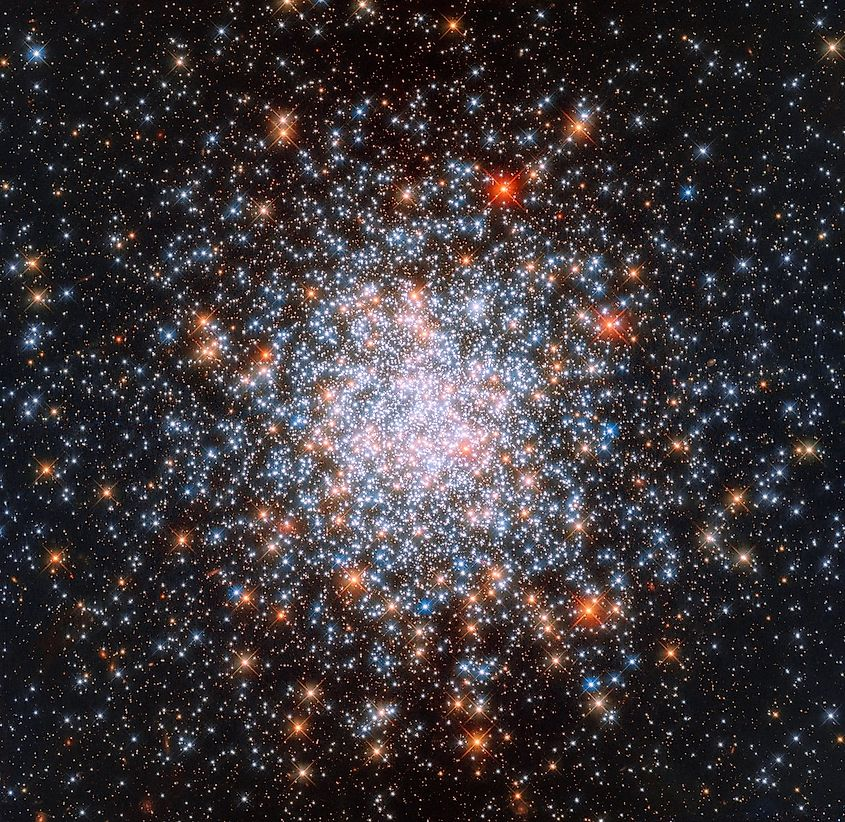# What Is The Drake Equation?

The Drake Equation was formulated by the astrophysicist Frank Drake in 1961. Drake’s goal in devising the equation was to come up with an approximate way for determining the number of planets in our galaxy that possibly contain intelligent alien life.

## Factors

The Drake Equation is as follows: N = R* x Fp x Ne x F1 x Fi x Fc x L. In this equation, N represents the number of intelligent civilizations in our galaxy that are capable of interstellar communication. All of the variables that come after represent various factors that are likely to influence the number of intelligent civilizations, and each value is multiplied by the other. R* represents the rate of star formation within the Milky Way. Fp is the number of those stars that have planets. Ne is the number of planets that can potentially develop habitable conditions. F1 is the number of those planets that actually do develop life. Fi is the number of those planets that end up producing intelligent life. Fc is the number of those planets where an intelligent civilization capable of interstellar communication develops, and L is the length of time those civilizations exist for. The equation itself is rather straightforward and makes sense, yet unfortunately, virtually none of these variables have known values. The only two that have estimates are R* and Fp. The current estimated rate of star formation in the Milky Way is around one to two stars a year. Interestingly, astronomers now believe that almost every star is accompanied by at least one planet. All the remaining variables remain unknown, and only Ne is likely to be determined in the near future.

## EstimatesThere are over 100-billion stars in our galaxy, most of which are likely accompanied by at least one planet. Image credit: NASA/ESA

Although most of the variables within the Drake Equation are unknown, that hasn’t stopped some scientists from making rough estimates of these variables to approximate the number of intelligent civilizations in our galaxy. Since the rate of star formation is known, as well as the average number of planets per star, only the remaining variables need approximations. In 1961, Frank Drake and his colleagues made an approximation of the Drake Equation. R* was estimated to be one, while Fp was estimated to be 0.5. For Ne, they estimated that every star that has planets will average at one to five planets being habitable, and that 100% of those planets will eventually develop both life and intelligent life. Then they estimated that 10% to 20% of those planets will develop a form of intelligent life capable of interstellar communication, and that they will exist for 1,000 years to 100-million years.

When the higher numbers are multiplied together, the value for N is 50-million. This means that by these estimates, the number of civilizations within our galaxy capable of interstellar communication is around 50-million.